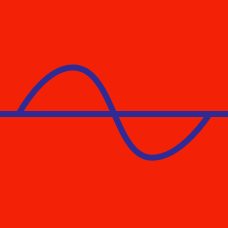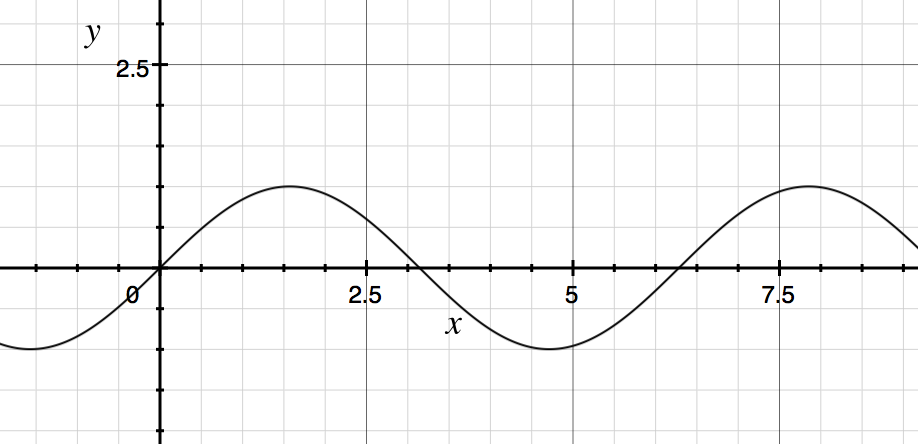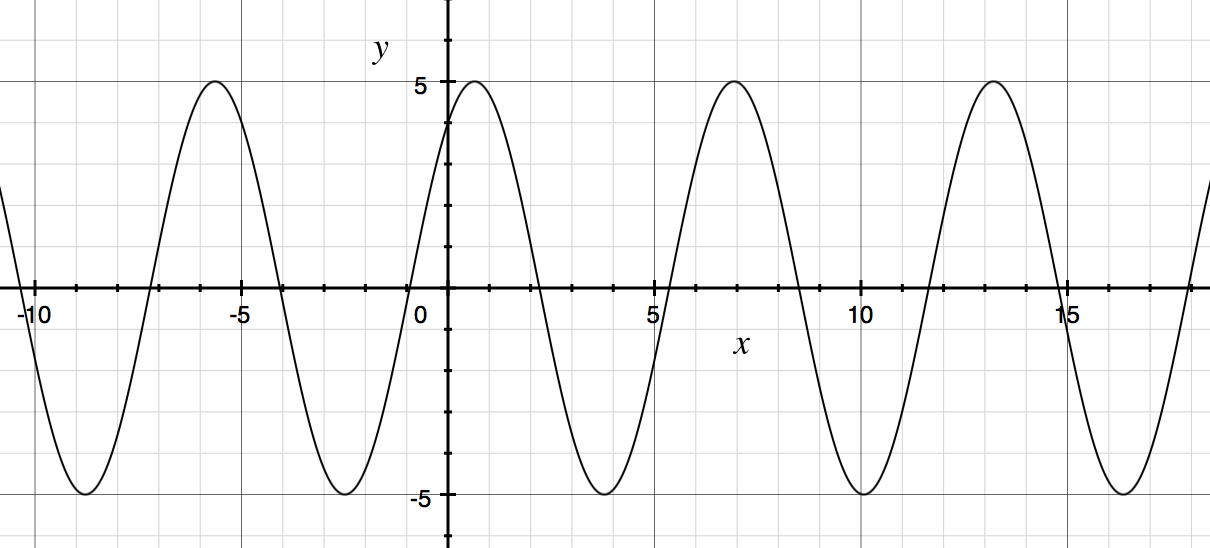Geometry

# Graphs of Trigonometric Functions: Level 2 ChallengesWhich of the following could be the function graphed above?

What is the amplitude of the graph of $\Large f(x) = \sin\left(x + \frac{\pi}{3}\right) + \cos\left(x+\frac{\pi}{6}\right)?$Given the graph above of $y = \color{#D61F06}{A}\sin(x)+\color{#3D99F6}{B}\cos(x)$ for integers $\color{#D61F06}{A}$ and $\color{#3D99F6}{B},$ find $\color{#D61F06}{A}-\color{#3D99F6}{B}.$

$\large \color{#69047E}{g(x)=\cos|x|+\sin|x|}$

Find the fundamental period of the function $\color{#69047E}{g(x)}$.

Find the fundamental period of the function $f(x) =\sin(36x)$.

×